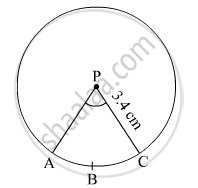# In the Given Figure, Radius of Circle is 3.4 Cm and Perimeter of Sector P-abc is 12.8 Cm . Find A(P-abc). - Geometry

#### Question

In the given figure, radius of circle is 3.4 cm and perimeter of sector P-ABC is 12.8 cm . Find A(P-ABC).#### Solution

Radius of the circle, r = 3.4 cm
Perimeter of sector P-ABC = 12.8 cm
Let l be the length of the arc ABC.
∴ l + 2r = 12.8 cm
⇒ l + 2 × 3.4 = 12.8
⇒ l = 12.8 − 6.8 = 6 cm
∴ A(P-ABC) = Area of the sector PABC = $\frac{1}{2}lr = \frac{1}{2} \times 6 \times 3 . 4$  = 10.2 cm2
Thus, A(P-ABC) is 10.2 cm2.

Is there an error in this question or solution?